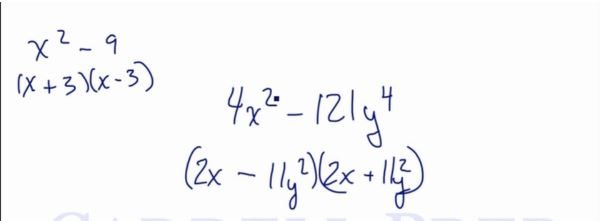In this video, we are going to look at how to understand the difference of perfect squares. After you finish this lesson, view all of our Algebra 1 lessons and practice problems.

For example:
When given something like$x^2-9$, we can rewrite it as$x^2+0x-9$
To factor this, we will need two numbers that add up to 0 and multiply to -9. These numbers are 3 and -3. Therefore when we factor this, we will get$(x+3)(x-3)$
Generally, whenever we have$x^2-b^2$ we will always be able to factor it as$(x+b)(x-b)$
Or$x^2-number$
will always be factored as$(x+\sqrt{number})(x-\sqrt{number})$
For expressions more complex, such as$4x^2-121y^4$
First, take the square root of the first term and place it in the front of each set of parentheses, and then take the square root of the second term and place it in the two parentheses with a plus or minus sign. This will be factored as$(2x-11y^2)(2x+11y^2)$Example of Difference Of Perfect Squares

Example 1

Factor$x^2-144$

will always be factored as$(x+\sqrt{number})(x-\sqrt{number})$$(x+\sqrt{144})(x-\sqrt{144})$
Therefore, this will be factored as$(x+12)(x-12)$

Factor$9x^2-81y^6$

First, take the square root of the first term and place it in front of each set of parentheses.
Then take the square root of the second term and place it in the two parentheses with a plus or minus sign.
Therefore, this will be factored as$(3x-9y^3)(3x+9y^3)$

Video-Lesson Transcript

Let’s go over the difference of perfect squares.

Let’s have some examples.$x^2 - 9$$x^2 - 16$$x^2 - 25$

The difference is associated with subtraction. That’s why we have a subtraction sign here.

Perfect squares are numbers that are the square root of.

The difference of perfect squares means two perfect squares to be subtracted.

Let’s take a look at how to factor these.$x^2 - 9$$x^2 + 0x - 9$$(x + 3) (x - 3)$

Next,$x^2 - 16$$x^2 + 0x - 16$$(x + 4) (x - 4)$

And$x^2 - 25$$x^2 + 0x - 25$$(x + 5) (x - 5)$

In general, the form is:$x^2 - b$$(x + b) (x - b)$

So if we have a perfect square$x^2 - \#$$(x + \sqrt{\#}) (x - \sqrt{\#})$

So if we have$x^2 - 81$$(x + 9) (x - 9)$

And of course, this can be switched into$(x - 9) (x + 9)$

It doesn’t make any difference.

This rule applies to all perfect squares.

For example:$4x^2 - 121y^4$

Let’s find the square root of the first term then the square root of the second term.$(2x - 11y^2) (2x + 11y^2)$

Look at this previous example:$x^2 - 9$

Let’s draw two parenthesis and put an$x$ inside each parenthesis.

If you solve that, the square root of$x^2$ is$x$.

Then the squareroot of$9$ is$3$.$(x + 3) (x - 3)$
So for$4x^2 - 121y^4$, we’ll find the squareroot of each term.
The squareroot of$4$ is$2$. The squareroot of$x^2$ is$x$. Then the squareroot of$121$ is$11$. Lastly, the squareroot of$y^4$ is$y^2$.